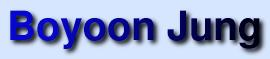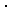Boyoon Jung's Library Manual

An adaptive particle filter class. More...

#include <particle.h>

Inherits bj::ParticleFilter.

Inherited by bj::ParticleMotionFilter.[legend][legend]
List of all members.

Public Member Functions

AdaptiveParticleFilter (int min_particles, int max_particles, int dimension, gsl_vector *min=0, gsl_vector *max=0, gsl_vector *bin_size=0)
A constructor.
A destructor.
virtual bool update (void)
Update particles.
double errorBound (void)
Return the current error bound.
void setErrorBound (double e)
set the new error bound.
double confidenceQuantile (void)
Return the current confidence quantile.
void setConfidenceQuantile (double z)
Set the new confidence quantile.
gsl_vector * binSize (void)
Return the current bin size.
void setBinSize (gsl_vector *bs)
Set the new bin size.
int min (void)
Return the lower bound of a state space.
int max (void)
Return the upper bound of a state space.
KDTreetree (void)
Return a kd-tree.

Protected Member Functions

int proper_size (int k)
Calculate the proper number of particles.

Protected Attributes

int min_size
The minimum number of particles.
int max_size
The maximum number of particles.
gsl_vector * bin_size
The size of bins for KD-tree.
KDTreekd_tree
A KD-tree.
double err_bound
Error bound.
double conf_quantile
Confidence quantile.

Constructor & Destructor Documentation

 bj::AdaptiveParticleFilter::AdaptiveParticleFilter ( int min_particles, int max_particles, int dimension, gsl_vector * min = 0, gsl_vector * max = 0, gsl_vector * bin_size = 0 )

A constructor.

Parameters:
 min_particles The minimum number of particles. max_particles The maximum number of particles. dimension The dimension of a particle. min The lower bound of the state space. max The upper bound of the state space. bin_size The size of bins for KD-tree.

 A destructor.

Member Function Documentation

 gsl_vector* bj::AdaptiveParticleFilter::binSize ( void ) [inline]
 Return the current bin size.

 double bj::AdaptiveParticleFilter::confidenceQuantile ( void ) [inline]
 Return the current confidence quantile.

 double bj::AdaptiveParticleFilter::errorBound ( void ) [inline]
 Return the current error bound.

 int bj::AdaptiveParticleFilter::max ( void ) [inline]
 Return the upper bound of a state space.

 int bj::AdaptiveParticleFilter::min ( void ) [inline]
 Return the lower bound of a state space.

 int bj::AdaptiveParticleFilter::proper_size ( int k ) [inline, protected]
 Calculate the proper number of particles.

 void bj::AdaptiveParticleFilter::setBinSize ( gsl_vector * bs ) [inline]
 Set the new bin size.

 void bj::AdaptiveParticleFilter::setConfidenceQuantile ( double z ) [inline]
 Set the new confidence quantile.

 void bj::AdaptiveParticleFilter::setErrorBound ( double e ) [inline]
 set the new error bound.

 KDTree* bj::AdaptiveParticleFilter::tree ( void ) [inline]
 Return a kd-tree.

 virtual bool bj::AdaptiveParticleFilter::update ( void ) [virtual]
 Update particles. Reimplemented from bj::ParticleFilter.

Member Data Documentation

 The size of bins for KD-tree.

 Confidence quantile.

 Error bound.

 A KD-tree.

 The maximum number of particles.

 The minimum number of particles.

The documentation for this class was generated from the following file:
ResearchProjectsPublications

ToolsBjLib ManualPlayer/StagePyPlayerMicroStrain IMUGPS/DGPSParticle Filter

PersonalWelcomeCurriculum VitaeResumeContactUSCUSC CS DeptCRESRobotics LabRESL